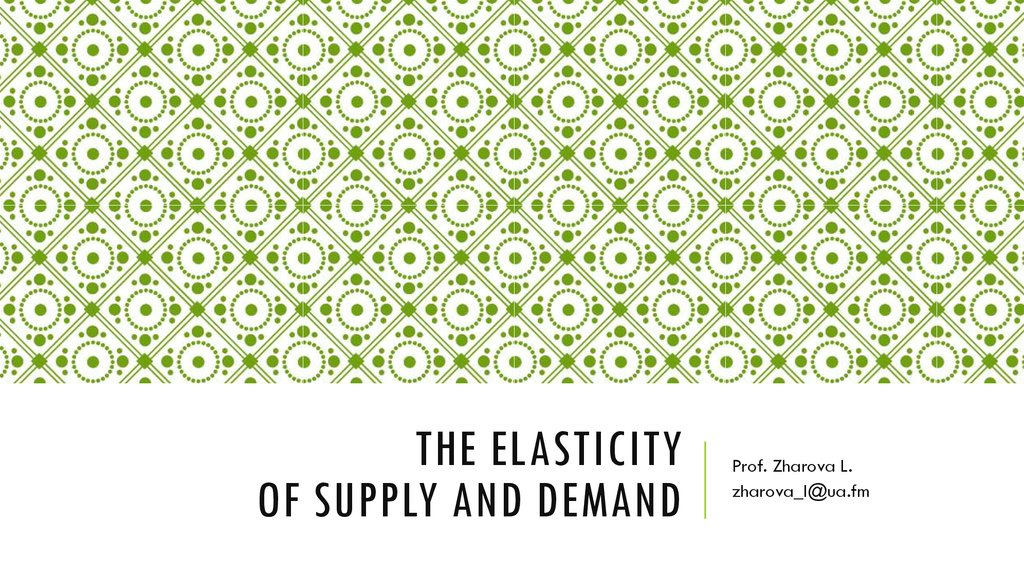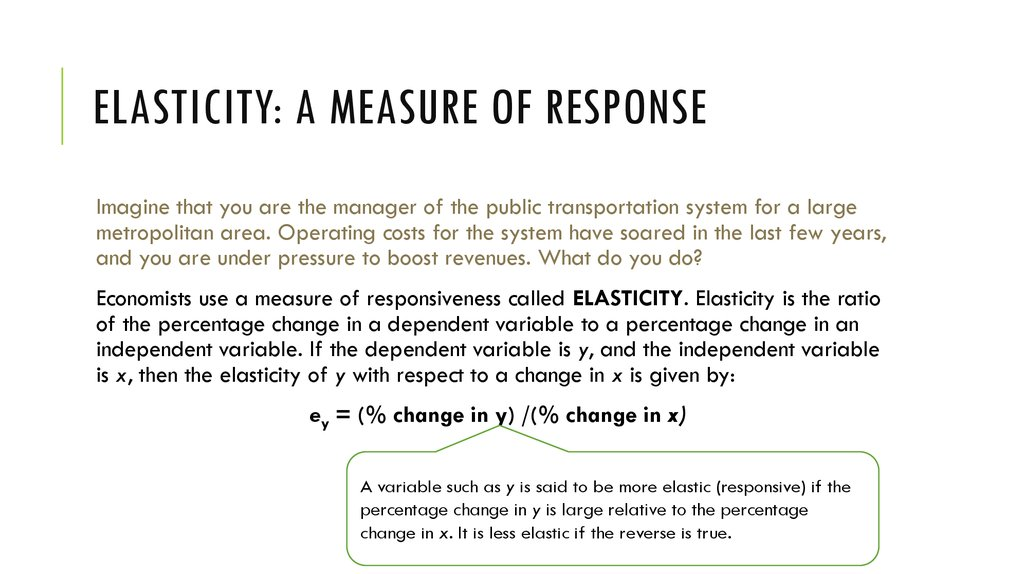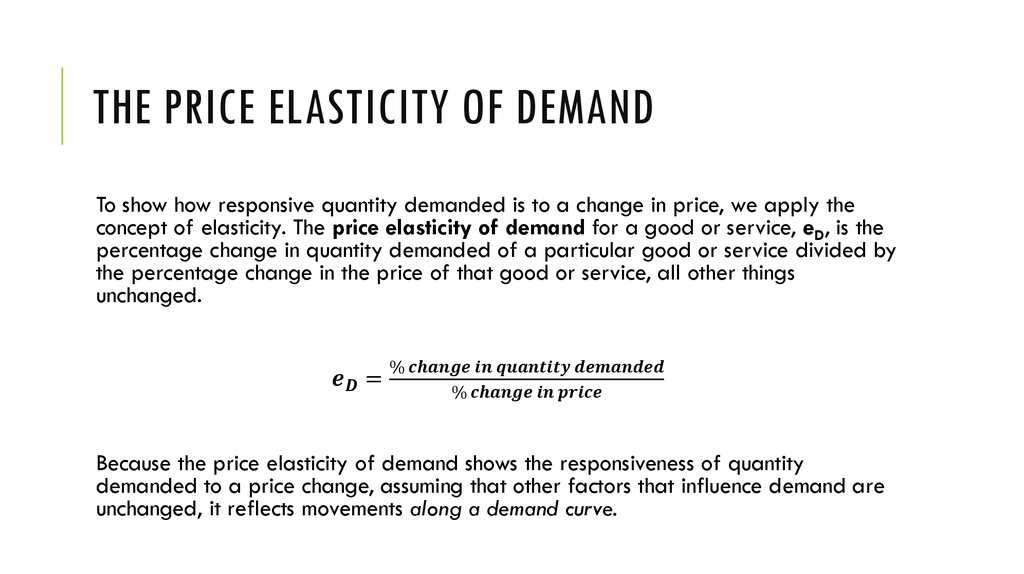# The Elasticity of supply and demand

## 1. The Elasticity of supply and demand

THE ELASTICITY
OF SUPPLY AND DEMAND
Prof. Zharova L.
[email protected]

## 2. Elasticity: A Measure of Response

ELASTICITY: A MEASURE OF RESPONSE
Imagine that you are the manager of the public transportation system for a large
metropolitan area. Operating costs for the system have soared in the last few years,
and you are under pressure to boost revenues. What do you do?
Economists use a measure of responsiveness called ELASTICITY. Elasticity is the ratio
of the percentage change in a dependent variable to a percentage change in an
independent variable. If the dependent variable is y, and the independent variable
is x, then the elasticity of y with respect to a change in x is given by:
ey = (% change in y) /(% change in x)
A variable such as y is said to be more elastic (responsive) if the
percentage change in y is large relative to the percentage
change in x. It is less elastic if the reverse is true.

## 3. The Price Elasticity of Demand

THE PRICE ELASTICITY OF DEMAND
To show how responsive quantity demanded is to a change in price, we apply the
concept of elasticity. The price elasticity of demand for a good or service, eD, is the
percentage change in quantity demanded of a particular good or service divided by
the percentage change in the price of that good or service, all other things
unchanged.